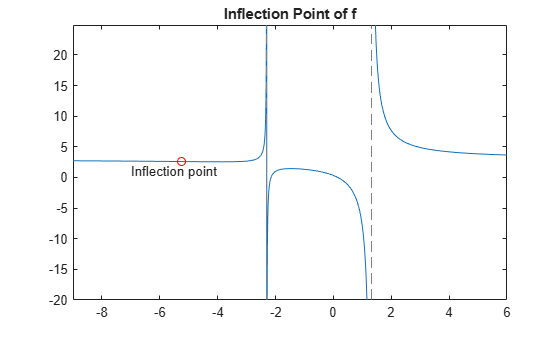# Find Asymptotes, Critical, and Inflection Points

This example describes how to analyze a simple function to find its asymptotes, maximum, minimum, and inflection point.

### Define a Function

The function in this example is

`$\mathit{f}\left(\mathit{x}\right)=\frac{3{\mathit{x}}^{2}+6\mathit{x}-1}{{\mathit{x}}^{2}+\mathit{x}-3}.$`

First, create the function.

```syms x num = 3*x^2 + 6*x -1; denom = x^2 + x - 3; f = num/denom```
```f =  $\frac{3 {x}^{2}+6 x-1}{{x}^{2}+x-3}$```

Plot the function by using `fplot`. The `fplot` function automatically shows vertical asymptotes.

`fplot(f)`### Find Asymptotes

To find the horizontal asymptote of $f$ mathematically, take the limit of $f$ as $x$ approaches positive infinity.

`limit(f,Inf)`
`ans = $3$`

The limit as $x$ approaches negative infinity is also 3. This result means the line $y=3$ is a horizontal asymptote to $f$.

To find the vertical asymptotes of $f$, set the denominator equal to 0 and solve it.

`roots = solve(denom)`
```roots =  $\left(\begin{array}{c}-\frac{\sqrt{13}}{2}-\frac{1}{2}\\ \frac{\sqrt{13}}{2}-\frac{1}{2}\end{array}\right)$```

`roots` indicates that the vertical asymptotes are the lines

`$\mathit{x}=\frac{-1-\sqrt{13}}{2}$`

and

$\mathit{x}=\frac{-1+\sqrt{13}}{2}$.

### Find Maximum and Minimum

You can see from the graph that $f$ has a local maximum between the points $x=–2$ and $x=0$. It also has a local minimum between $x=–6$ and $x=–2$. To find the $x$-coordinates of the maximum and minimum, first take the derivative of $f$.

`f1 = diff(f)`
```f1 =  $\frac{6 x+6}{{x}^{2}+x-3}-\frac{\left(2 x+1\right) \left(3 {x}^{2}+6 x-1\right)}{{\left({x}^{2}+x-3\right)}^{2}}$```

To simplify this expression, enter the following.

`f1 = simplify(f1)`
```f1 =  $-\frac{3 {x}^{2}+16 x+17}{{\left({x}^{2}+x-3\right)}^{2}}$```

Next, set the derivative equal to 0 and solve for the critical points.

`crit_pts = solve(f1)`
```crit_pts =  $\left(\begin{array}{c}-\frac{\sqrt{13}}{3}-\frac{8}{3}\\ \frac{\sqrt{13}}{3}-\frac{8}{3}\end{array}\right)$```

As the graph of $f$ shows, the function has a local minimum at

`${\mathit{x}}_{1}=\frac{-8-\sqrt{13}}{3}$`

and a local maximum at

${\mathit{x}}_{1}=\frac{-8+\sqrt{13}}{3}$.

Plot the maximum and minimum of `f`.

```fplot(f) hold on plot(double(crit_pts), double(subs(f,crit_pts)),'ro') title('Maximum and Minimum of f') text(-4.8,5.5,'Local minimum') text(-2,4,'Local maximum') hold off```### Find Inflection Point

To find the inflection point of $f$, set the second derivative equal to 0 and solve for this condition.

```f2 = diff(f1); inflec_pt = solve(f2,'MaxDegree',3); double(inflec_pt)```
```ans = 3×1 complex -5.2635 + 0.0000i -1.3682 - 0.8511i -1.3682 + 0.8511i ```

In this example, only the first element is a real number, so this is the only inflection point. MATLAB® does not always return the roots to an equation in the same order.

Instead of selecting the real root by indexing into `inter_pt`, identify the real root by determining which roots have a zero-valued imaginary part.

```idx = imag(double(inflec_pt)) == 0; inflec_pt = inflec_pt(idx)```
```inflec_pt =  $-\frac{13}{9 {\left(\frac{169}{54}-\frac{\sqrt{2197}}{18}\right)}^{1/3}}-{\left(\frac{169}{54}-\frac{\sqrt{2197}}{18}\right)}^{1/3}-\frac{8}{3}$```

Plot the inflection point. The extra argument `[-9 6]` in `fplot` extends the range of $x$ values in the plot so that you can see the inflection point more clearly, as the figure shows.

```fplot(f,[-9 6]) hold on plot(double(inflec_pt), double(subs(f,inflec_pt)),'ro') title('Inflection Point of f') text(-7,1,'Inflection point') hold off```## Support

#### Mathematical Modeling with Symbolic Math Toolbox

Get examples and videos